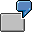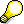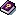Formula Variables in ReportsA formula variable represents a user-defined formula, which determines a value for the variable. In contrast to a value variable, the default value for a formula variable is not fixed. When you execute a report that uses formula variables, the system calculates values for the formula variables automatically (for example, using the system date or other variables).• The Report Writer only generates input fields for independent variables on the report selection screen when you execute the report’s report group. An independent variable does not depend on the value of another variable. You should define your variables so that you only have to enter independent values on the report selection screen.

For example, your report displays data for the current year and the previous year. If you create two independent variables for the current and previous year, you have to enter both years on the selection screen when you output the report. If you define the variable for the previous year as a formula variable, which derives its value from the current year variable, you only have to enter the current year on the selection screen.

• You do not have to use independent variables in the actual report definition. If a report only uses dependent variables, the Report Writer determines the values of the independent variables and creates input fields for these variables on the report selection screen.

For more information on creating formula variables, seeCreating Formula Variables.

Using formula variables, you can create several new types of reports. The following examples illustrate how you can use formula variables in your reports.

Example 1: Rolling Periods

See the example report 0R-FOVAR (library 0R1) delivered with the Special Purpose Ledger (FI-SL) application component.

This report uses formula variables to create report columns containing twelve periods. When you enter the reporting period and the reporting year during report execution, the system outputs data for the reporting period and the previous eleven periods.

To define the example report with rolling periods, you need to create twelve formula variables for the twelve periods, and twelve formula variables for the corresponding fiscal year. You should create the variables in the order listed in the table below.

You create a key figure for each pair of variables when you create a Report Writer report. (You must first create basic and multidimension sets that contain the formula variables.) Alternatively, you can enter the variables directly in the report definition if you are creating a Report Painter report.

Formula Variables Used in the Example Rolling Periods Report

 Period variable Formula Year variable Formula Column 12 &0R-RP00 ‘#S002’ &0R-RY00 ‘#S001’ Column 11 &0R-RP01 IF ‘0R-RP00’ > 1 THEN ‘0R-RP00’ - 1 ELSE 12 &0R-RY01 IF ‘0R-RP01’ < ‘0R-RP00’ THEN ‘0R-RY00’ ELSE ‘0R-RY00’ - 1 Column 10 &0R-RP02 IF ‘0R-RP01’ > 1 THEN ‘0R-RP01’ - 1 ELSE 12 &0R-RY02 IF ‘0R-RP02’ < ‘0R-RP01’ THEN ‘0R-RY01’ ELSE ‘0R-RY01’ - 1 Column 9 &0R-RP03 IF ‘0R-RP02’ > 1 THEN ‘0R-RP02’ - 1 ELSE 12 &0R-RY03 IF ‘0R-RP03’ < ‘0R-RP02’ THEN ‘0R-RY02’ ELSE ‘0R-RY02’ - 1 Column 8 &0R-RP04 IF ‘0R-RP03’ > 1 THEN ‘0R-RP03’ - 1 ELSE 12 &0R-RY04 IF ‘0R-RP04’ < ‘0R-RP03’ THEN ‘0R-RY03’ ELSE ‘0R-RY03’ - 1 Column 7 &0R-RP05 IF ‘0R-RP04’ > 1 THEN ‘0R-RP04’ - 1 ELSE 12 &0R-RY05 IF ‘0R-RP05’ < ‘0R-RP04’ THEN ‘0R-RY04’ ELSE ‘0R-RY04’ - 1 Column 6 &0R-RP06 IF ‘0R-RP05’ > 1 THEN ‘0R-RP05’ - 1 ELSE 12 &0R-RY06 IF ‘0R-RP06’ < ‘0R-RP05’ THEN ‘0R-RY05’ ELSE ‘0R-RY05’ - 1 Column 5 &0R-RP07 IF ‘0R-RP06’ > 1 THEN ‘0R-RP06’ - 1 ELSE 12 &0R-RY07 IF ‘0R-RP07’ < ‘0R-RP06’ THEN ‘0R-RY06’ ELSE ‘0R-RY06’ - 1 Column 4 &0R-RP08 IF ‘0R-RP07’ > 1 THEN ‘0R-RP07’ - 1 ELSE 12 &0R-RY08 IF ‘0R-RP08’ < ‘0R-RP07’ THEN ‘0R-RY07’ ELSE ‘0R-RY07’ - 1 Column 3 &0R-RP09 IF ‘0R-RP08’ > 1 THEN ‘0R-RP08’ - 1 ELSE 12 &0R-RY09 IF ‘0R-RP09’ < ‘0R-RP08’ THEN ‘0R-RY08’ ELSE ‘0R-RY08’ - 1 Column 2 &0R-RP10 IF ‘0R-RP09’ > 1 THEN ‘0R-RP09’ - 1 ELSE 12 &0R-RY10 IF ‘0R-RP10’ < ‘0R-RP09’ THEN ‘0R-RY09’ ELSE ‘0R-RY09’ - 1 Column 1 &0R-RP11 IF ‘0R-RP10’ > 1 THEN ‘0R-RP10’ - 1 ELSE 12 &0R-RY11 IF ‘0R-RP11’ < ‘0R-RP10’ THEN ‘0R-RY10’ ELSE ‘0R-RY10’ - 1

The formula variables &0R-RP00 (period of the last column) and &0R-RY00 (fiscal year of the last column) are the only independent variables in the report columns. Each pair of variables calculates the period and fiscal year of the preceding period. The year changes when the system calculates the preceding periods based on period one.

Example 2: Displaying Periods of Quarter q

This example report displays three columns for the periods of quarter q.

To define the report columns, you must first create the value variable &QUARTER for the characteristic Period. You enter Quarter as the variable description. This variable is the independent variable. You then need to create three formula variables (listed in the table below) for the three periods in the quarter.

Formula Variables Used to Calculate the Periods in Quarter q

 Period variable Formula Column 1 &QPER1 ‘QUARTER’ * 3 - 2 Column 2 &QPER2 ‘QUARTER’ * 3 - 1 Column 3 &QPER3 ‘QUARTER’ * 3

The above example assumes that the first quarter consists of periods one, two, and three. You enter the formula variables &QPER1, &QPER2 and &QPER3 in key figures if you are creating a Report Writer report. Alternatively, you can enter the variables directly in the column definition if you are creating a Report Painter report.

The independent variable &QUARTER is not used directly in the report; it is used indirectly via the formula variables. When you execute the report’s report group, the Report Writer creates the input field Quarter for the independent variable &QUARTER. You can then enter quarter one, two, three, or four to output your report for the respective quarter.

Example 3: Displaying Periods of Quarter q Based on Period p

This example report displays three columns for the periods of quarter q. Unlike the report in example two, you enter one period in the quarter and not the quarter itself on the report selection screen to determine the three period columns.

To define the report columns, you must first create the value variable &PERIOD for the characteristic Period. Enter Period as the variable name. This variable is the independent variable. You then need to create three formula variables (listed in the table below), which determine the three periods in the quarter.• The DIV operator in the formulas denotes division without remainder (for example, 8 DIV 3 = 2).
• The system processes the formula enclosed in parentheses first to determine the quarter based on the period you enter on the report selection screen. It then processes the rest of the formula to calculate the periods in the first quarter.

Formula Variables Used to Calculate the Periods of Quarter q Based on Period p

 Period variable Formula Column 1 &QPER1 ((‘PERIOD’ - 1) DIV 3 + 1) * 3 - 2 Column 2 &QPER2 ((‘PERIOD’ - 1) DIV 3 + 1) * 3 - 1 Column 3 &QPER3 ((‘PERIOD’ - 1) DIV 3 + 1) * 3

The above example assumes that the first quarter consists of periods one, two, and three. The independent variable &PERIOD is not used directly in the report; it is used indirectly via the formula variables. When you execute the report’s report group, the Report Writer creates the input field Period for the independent variable &PERIOD. You can then enter period one, two or three to output your report for the periods in the first quarter.

Example 4: Displaying a Variable Number of Columns for Periods p1 to p12

This example report allows you to create a report containing a variable number of columns for periods one to twelve. It is not possible to define a variable number of columns directly in the report definition; you can do so, however, by creating a column block consisting of twelve columns, each represented by a formula variable.The formula variables map the unwanted periods up to period 16. If data has been posted for period 16 in your system, you should use a different period that does not contain data (for example, period 17).

To define the report columns, you must first create the value variables &PER_FROM and &PER_TO for the characteristic Period. These variables are the independent variables. You must then create the formula variables (listed in the table below), which all refer to the independent period variables. Once you have created the formula variables, you must enter them in a basic set (created for the characteristic Period) if you are creating a Report Writer report. Alternatively, you can enter them directly in the column definition if you are creating a Report Painter report.

Formula Variables Used to Display a Variable Number of Report Columns

 Period variable Formula Column 1 &PER01 IF 1 >= ‘PER_FROM’ AND 1 <= ‘PER_TO’ THEN 1 ELSE 16 Column 2 &PER02 IF 2 >= ‘PER_FROM’ AND 2 <= ‘PER_TO’ THEN 2 ELSE 16 Column 3 &PER03 IF 3 >= ‘PER_FROM’ AND 3 <= ‘PER_TO’ THEN 3 ELSE 16 Column 4 &PER04 IF 4 >= ‘PER_FROM’ AND 4 <= ‘PER_TO’ THEN 4 ELSE 16 Column 5 &PER05 IF 5 >= ‘PER_FROM’ AND 5 <= ‘PER_TO’ THEN 5 ELSE 16 Column 6 &PER06 IF 6 >= ‘PER_FROM’ AND 6 <= ‘PER_TO’ THEN 6 ELSE 16 Column 7 &PER07 IF 7 >= ‘PER_FROM’ AND 7 <= ‘PER_TO’ THEN 7 ELSE 16 Column 8 &PER08 IF 8 >= ‘PER_FROM’ AND 8 <= ‘PER_TO’ THEN 8 ELSE 16 Column 9 &PER09 IF 9 >= ‘PER_FROM’ AND 9 <= ‘PER_TO’ THEN 9 ELSE 16 Column 10 &PER10 IF 10 >= ‘PER_FROM’ AND 10 <= ‘PER_TO’ THEN 10 ELSE 16 Column 11 &PER11 IF 11 >= ‘PER_FROM’ AND 11 <= ‘PER_TO’ THEN 11 ELSE 16 Column 12 &PER12 IF 12 >= ‘PER_FROM’ AND 12 <= ‘PER_TO’ THEN 12 ELSE 16The Suppress zero columns indicator must be set in the standard layout assigned to the report, so that columns that do not contain any values are not displayed in the report output.

When you execute the report’s report group, the Report Writer creates input fields for the independent variables &PER_FROM and &PER_TO, which determine the period range.

Example 5: Calculating Defaults for a Non-Calendar Fiscal Year

You can create formula variables that default to the current period and fiscal year taken from the system date. If you work with a non-calendar fiscal year, you can create formula variables that calculate the correct period and fiscal year using the current system date and period.

Example Formula Variables to Calculate Defaults for a Non-Calendar Fiscal Year

 Description Formula Calendar year from the system date ‘#S001’ Calendar month from the system date ‘#S002’ Non-calendar fiscal year calculated from the system date (the non-calendar fiscal year starts in July) IF ‘#S002’ > 6 THEN ‘#S001’ + 1 ELSE ‘#S001’ Period calculated from the system date (the non-calendar fiscal year starts in July) IF ‘#S002’ >6 THEN ‘#S002’ - 6 ELSE ‘#002’ + 6